SLVSE57C June   2017  – April 2018

PRODUCTION DATA.

1. Features
2. Applications
3. Description
1.     Device Images
4. Revision History
5. Device Comparison Table
6. Pin Configuration and Functions
7. Specifications
8. Detailed Description
1. 8.1 Overview
2. 8.2 Functional Block Diagram
3. 8.3 Feature Description
4. 8.4 Device Functional Modes
9. Application and Implementation
1. 9.1 Application Information
2. 9.2 Typical Application
10. 10Power Supply Recommendations
11. 11Layout
12. 12Device and Documentation Support
13. 13Mechanical, Packaging, and Orderable Information

• DSG|8
• DSG|8

### 7.7 Typical Characteristics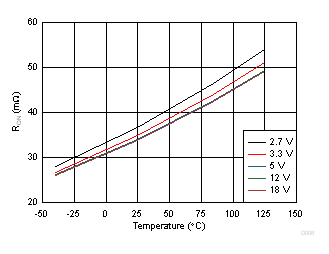IOUT = 0.5A , Different Input Voltages
Figure 3. On-resistance vs TemperatureTPS2595x5
Figure 5. UVP Threshold vs Temperature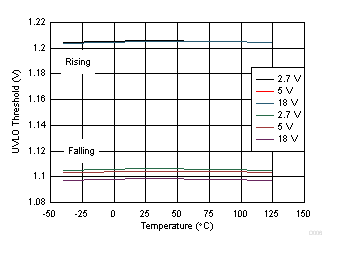TPS2595x0/1/5, Different Input Voltages
Figure 7. UVLO Threshold vs Temperature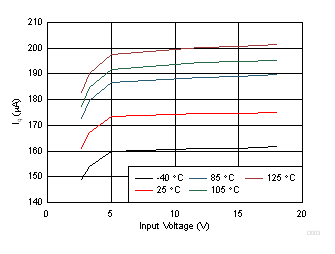Figure 9. Quiescent Current vs Input Voltage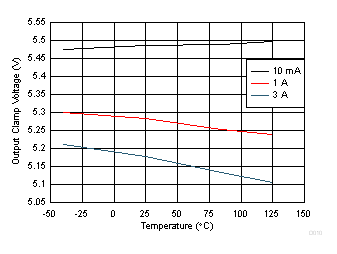Figure 11. OVC Threshold vs Temperature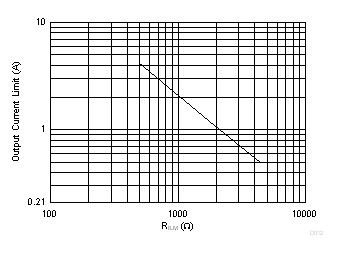Figure 13. Output Current Limit (ILIMIT) vs RILM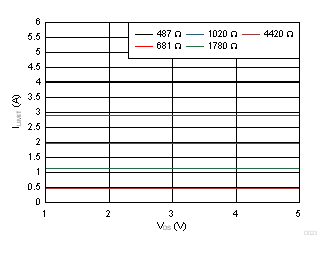Different RILM values
Figure 15. Output Current Limit vs VDS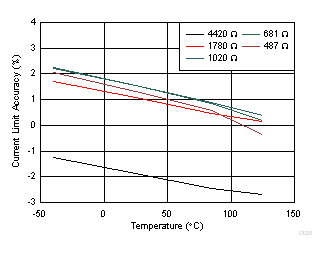VIN = 3.3 V, Different RILM values
Figure 17. Output Current Limit Accuracy vs Temperature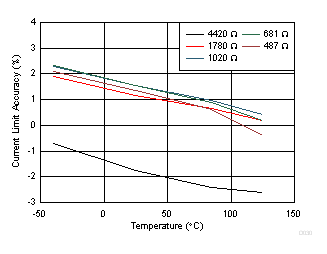VIN = 12 V, Different RILM values
Figure 19. Output Current Limit Accuracy vs Temperature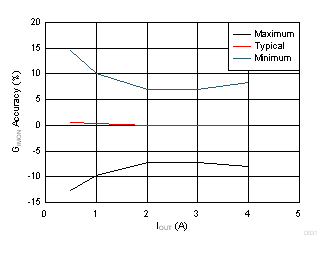Normalized to reference value of GIMON = 276uA/A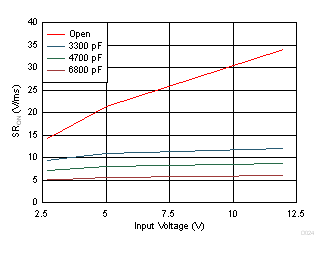Different CdVdt values
Figure 23. ON Slew Rate vs Input Voltage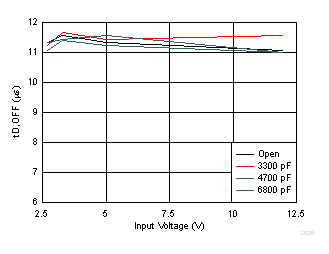Different CdVdt values
Figure 25. Turn OFF Delay vs Input Voltage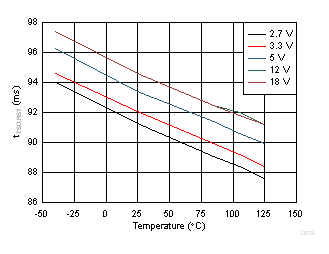TPS2595x1/3/5, Different Input Voltages
Figure 27. Thermal Shutdown Auto-Retry Interval vs Temperature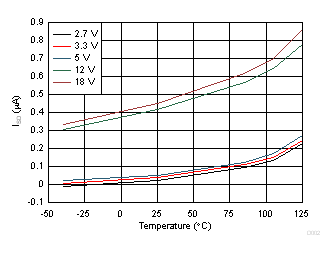TPS2595x0/1/5, EN=GND, OUT=Open, Different Input Voltages
Figure 4. Shutdown Current vs Temperature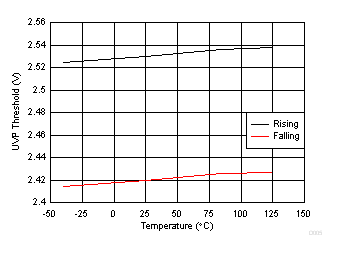TPS2595x0/1/3
Figure 6. UVP Threshold vs Temperature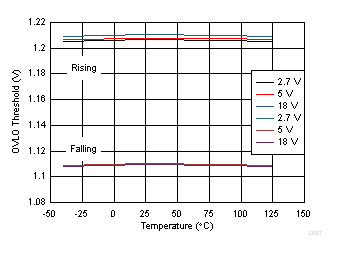TPS259573, Different Input Voltages
Figure 8. OVLO Threshold vs Temperature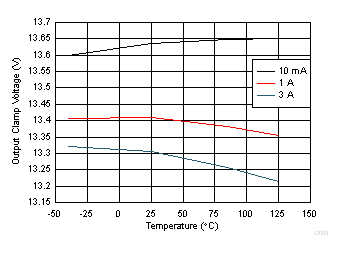Figure 10. OVC Threshold vs Temperature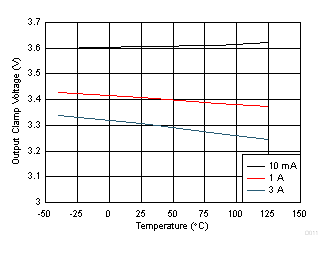Figure 12. OVC Threshold vs Temperature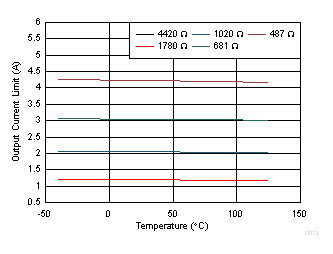VIN = 12V, Different RILM values
Figure 14. Output Current Limit (ILIMIT) vs TemperatureVIN = 5 V
Figure 16. Output Current Limit Accuracy vs Output Current Limit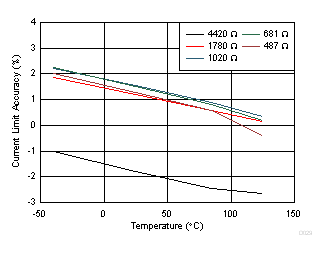VIN = 5 V, Different RILM values
Figure 18. Output Current Limit Accuracy vs Temperature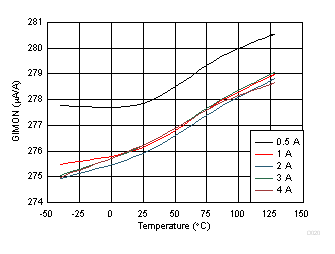Figure 20. Load Current Monitor Gain vs Temperature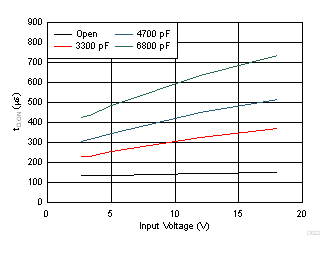Different CdVdt values
Figure 22. Turn on Delay vs Input Voltage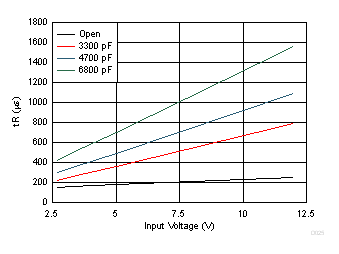Different CdVdt values
Figure 24. Rise Time vs Input VoltageFigure 26. Thermal Shutdown Time vs Power Dissipation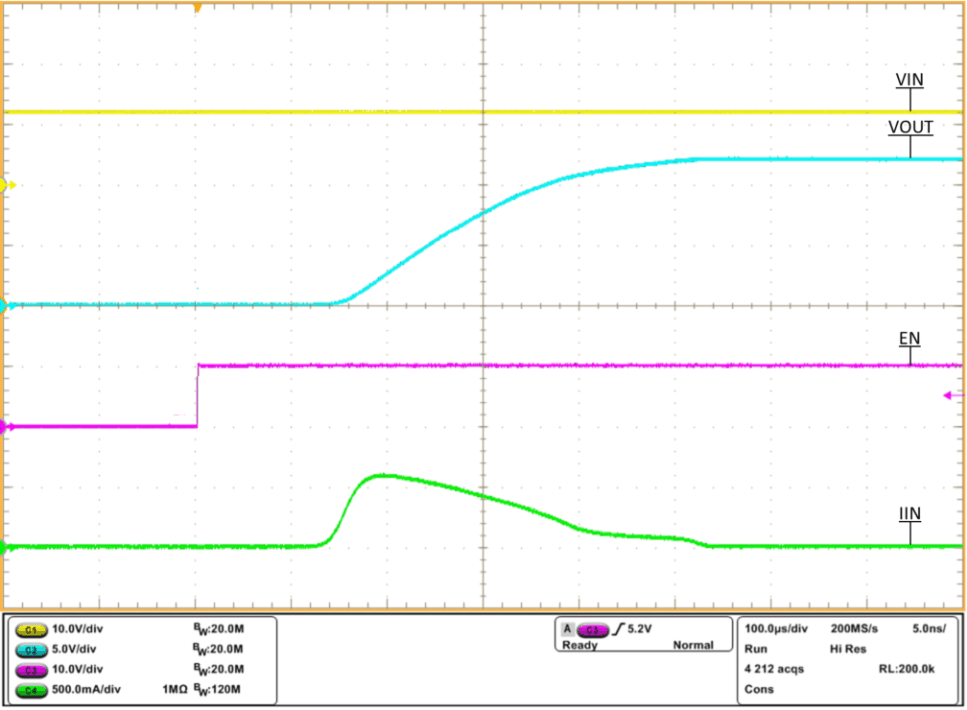VIN = 12 V, COUT = 10 µF, RILM = 487 Ω, VEN = 5 V
Figure 28. Output Voltage Ramp and Inrush Current at Start Up, CdVdT = Open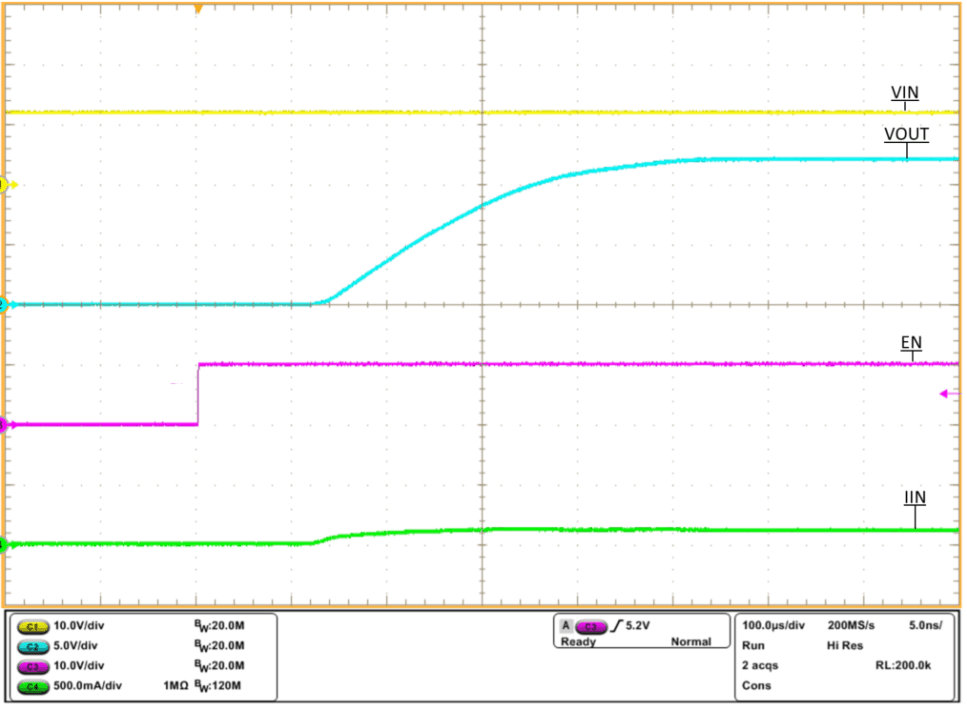VIN = 12 V, COUT = 1 µF, RILM = 487 Ω, ROUT = 100 Ω
Figure 30. Turn ON Delay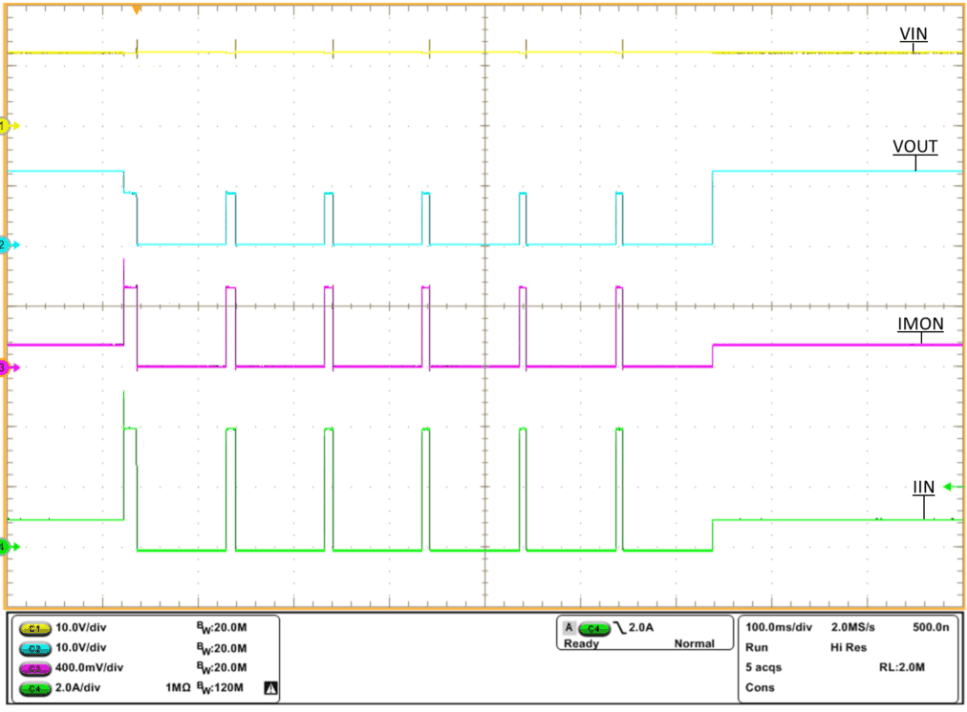VIN = 12 V, COUT = 1 µF, RILM = 487 Ω, ROUT varied from 12 Ω to 2 Ω to 12 Ω
Figure 32. TPS2595x1, TPS2595x3, TPS2595x5 OverCurrent Response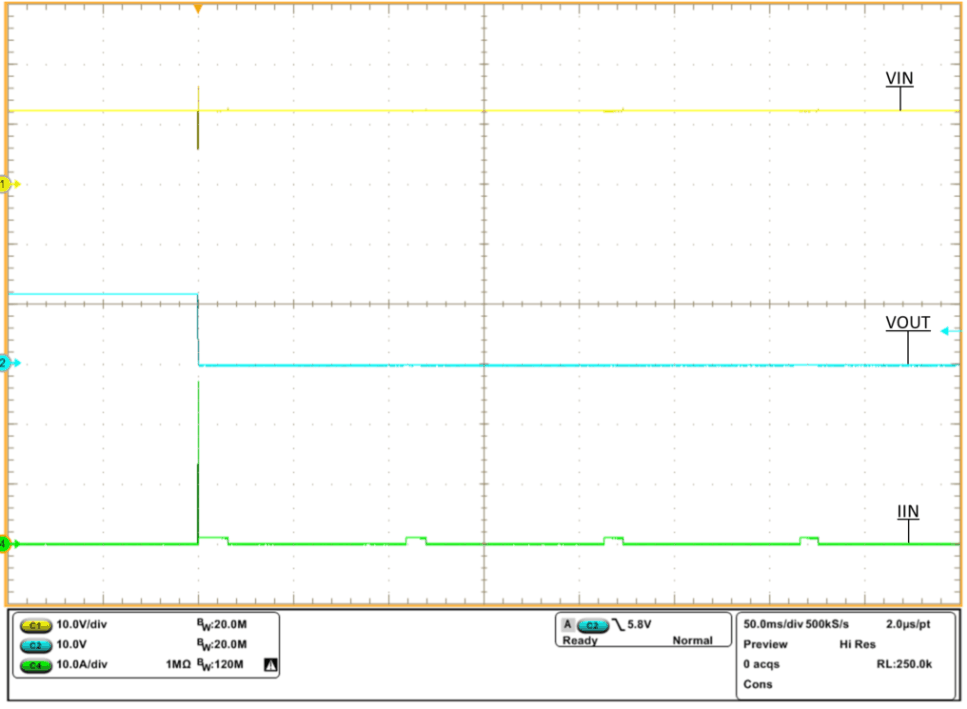VIN = 12 V, COUT = 1 µF, RILM = 487 Ω
Figure 34. Output Hot Short to GND Response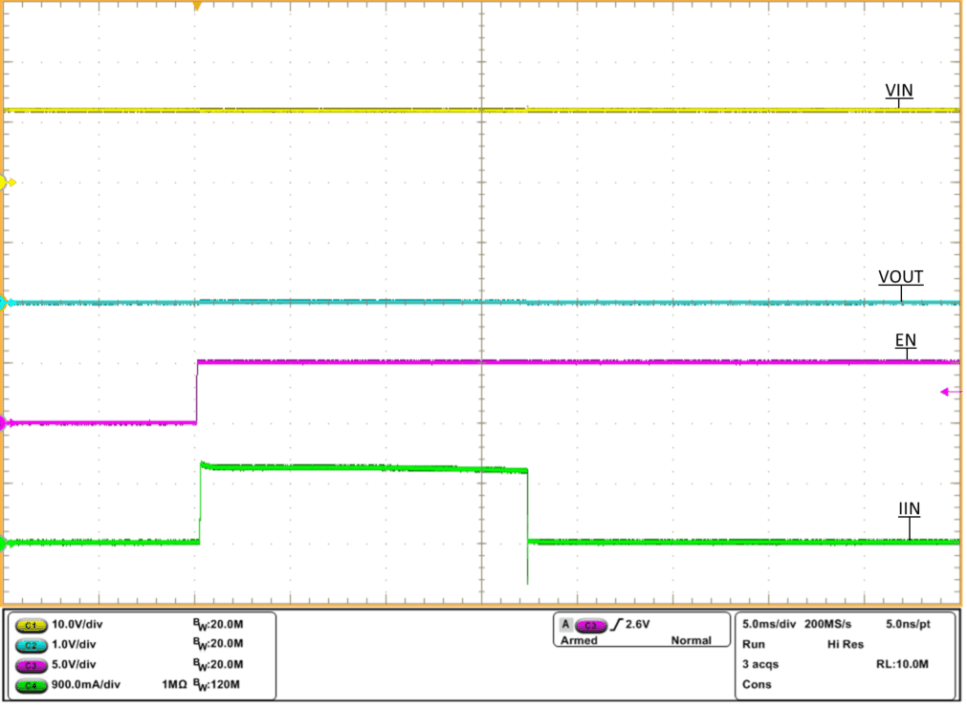VIN = 12 V, COUT = 1 µF, RILM = 487 Ω
Figure 36. Wake Up With Output Short to GND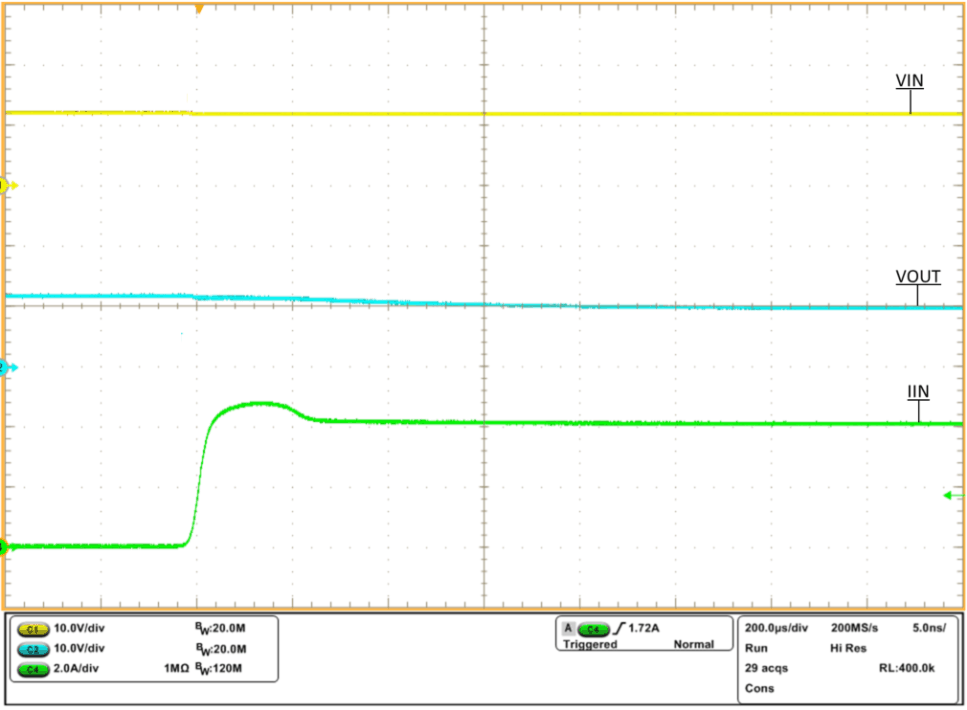VIN = 12 V, COUT = 1 µF, RILM = 487 Ω, IOUT stepped from 4 A to 4.8 A
Figure 38. Output Load Transient Response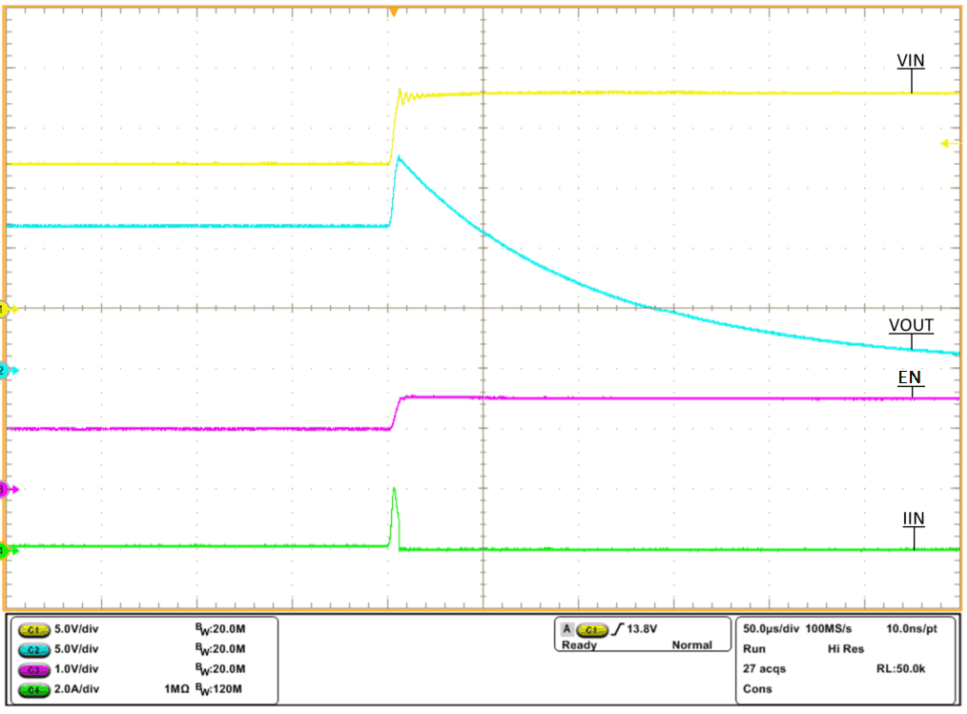VIN stepped from 12 V to 18 V, COUT = 1 µF, RILM = 487 Ω, FLT = 3.3 V through 10 kΩ, ROUT = 100 Ω
Figure 40. TPS259573 Overvoltage Lockout Response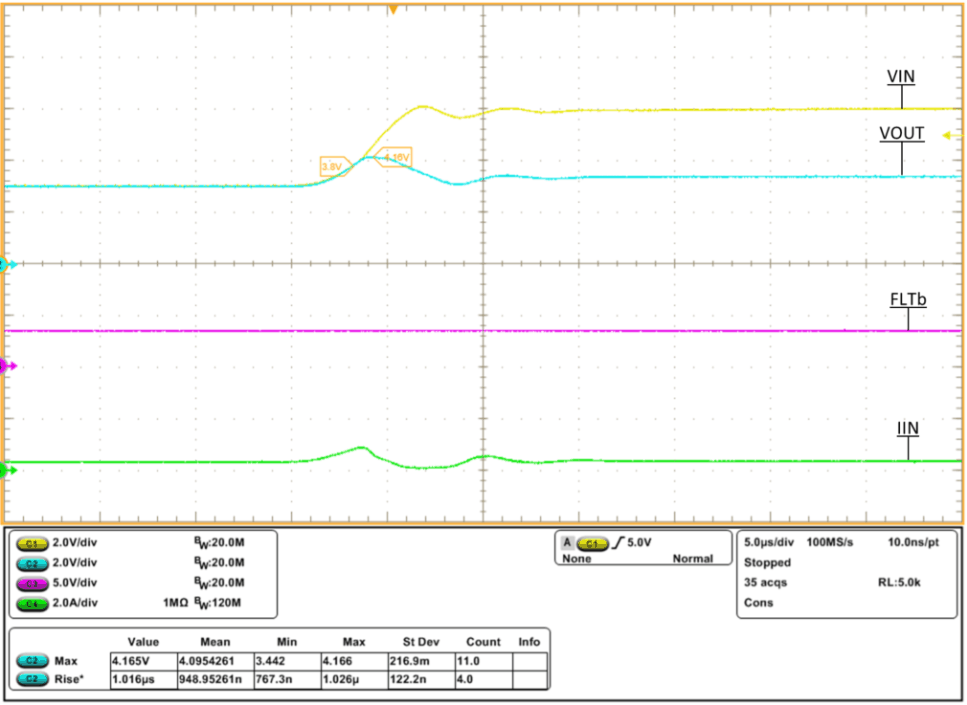VIN stepped from 3 V to 6 V, COUT = 1 µF, RILM = 487 Ω, FLT= 3.3 V through 10 kΩ, ROUT = 10 Ω
Figure 42. TPS25952x Overvoltage Clamp Response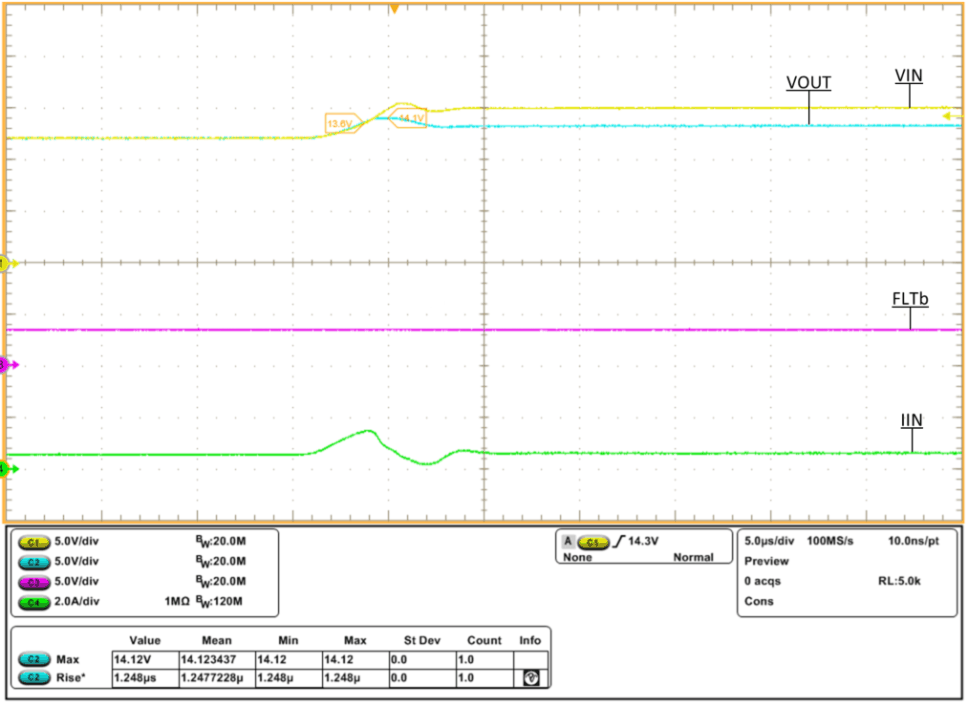VIN Stepped from 12 V to 15 V, COUT = 1 µF, RILM = 487 Ω, FLT = 3.3 V through 10 kΩ, ROUT = 20 Ω
Figure 44. TPS25954x Overvoltage Clamp Response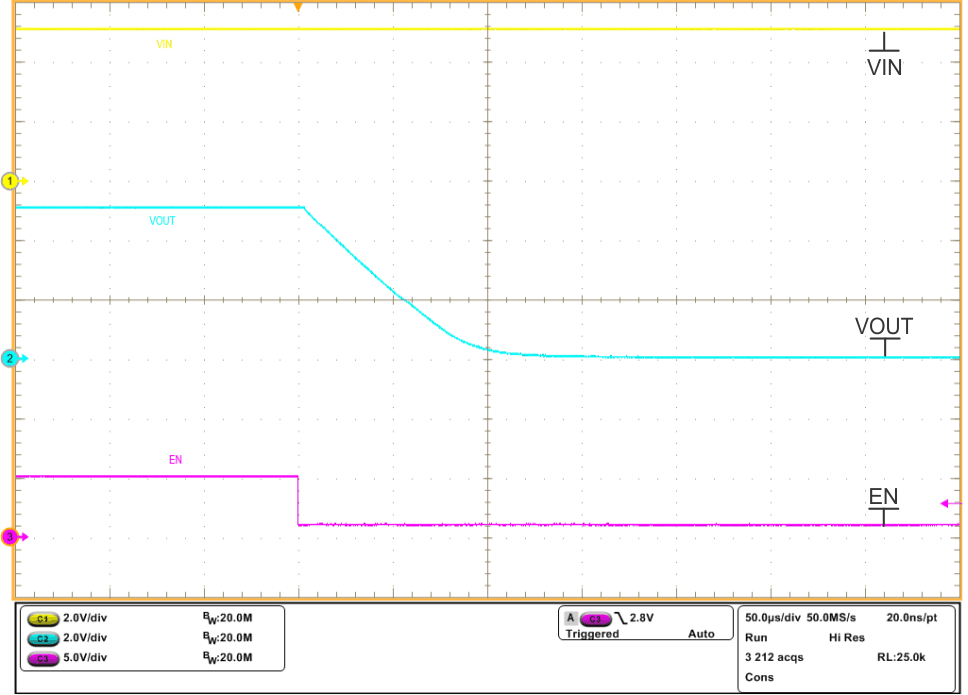VIN = 5 V, COUT = 1 µF, RILM = 487 Ω
Figure 46. TPS2595x5 Quick Output Discharge, EN stepped from 5 V to 1 VVIN = 12 V, COUT = 10 µF, RILM = 487 Ω, VEN = 5 V
Figure 29. Output Voltage Ramp and Inrush Current at Start Up, CdVdT = 22 nF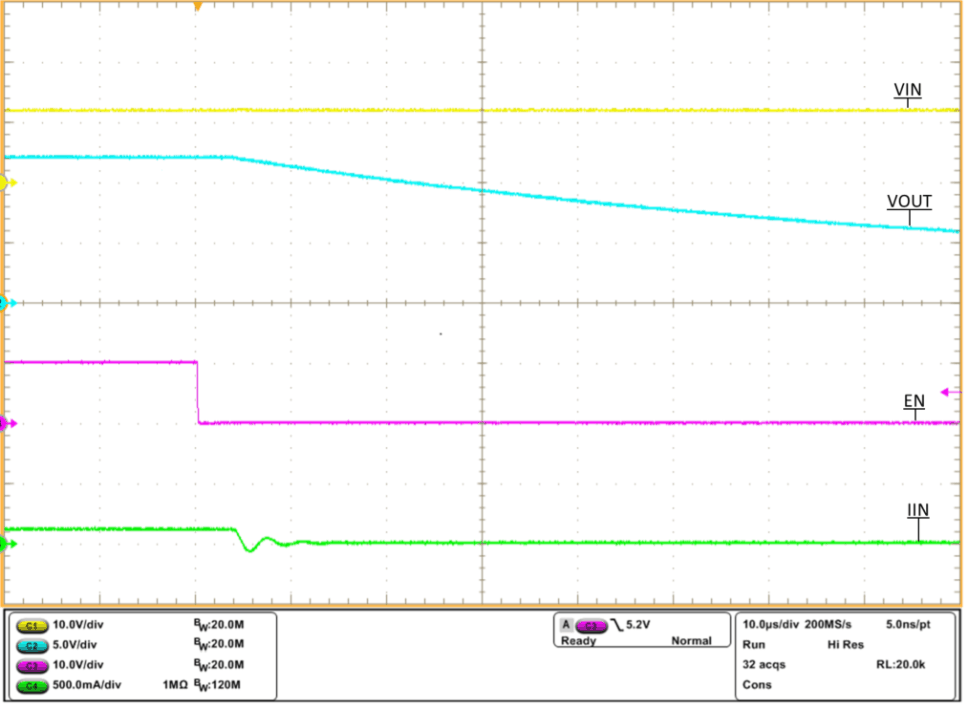VIN = 12 V, COUT = 1 µF, RILM = 487 Ω, ROUT = 100 Ω
Figure 31. Turn OFF Delay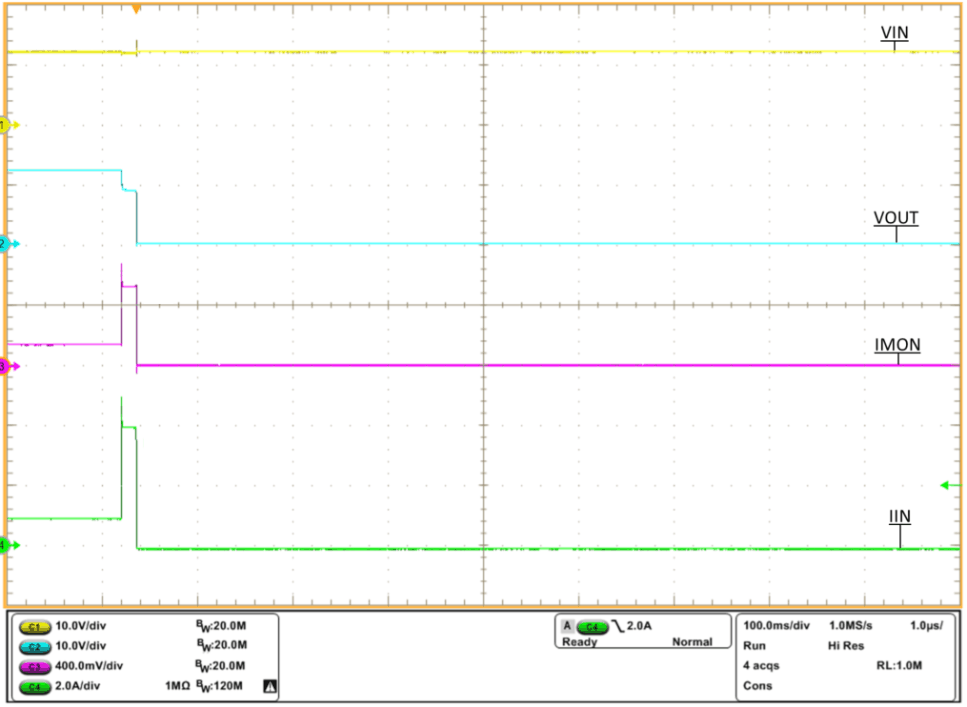VIN = 12 V, COUT = 1 µF, RILM = 487 Ω, ROUT varied from 12 Ω to 2 Ω to 12 Ω
Figure 33. TPS2595x0 Overcurrent Response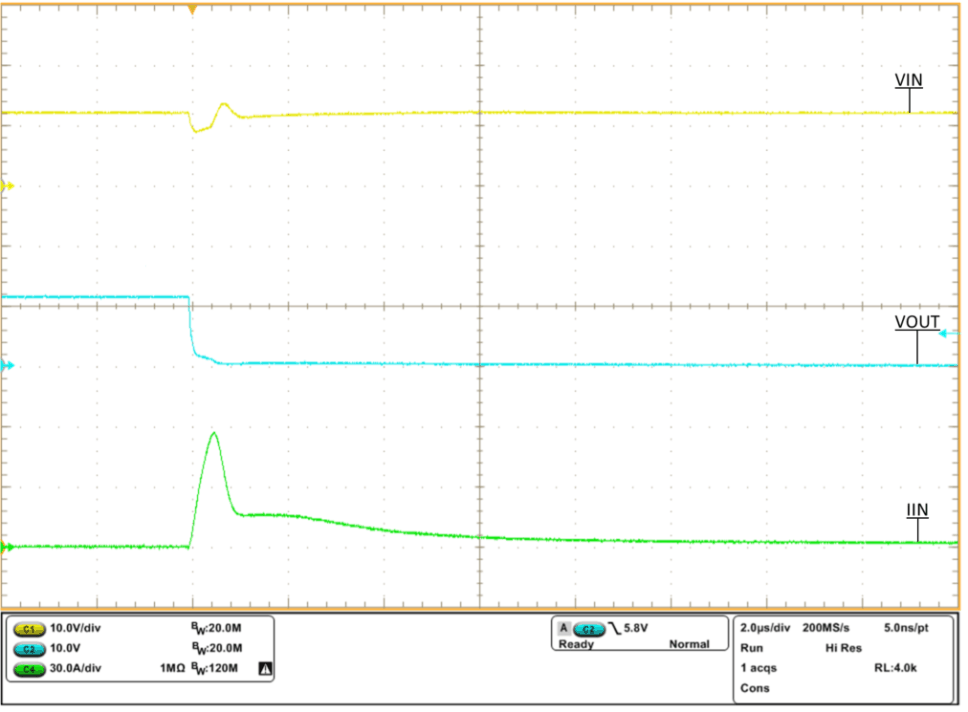VIN = 12 V, COUT = 1 µF, RILM = 487 Ω
Figure 35. Output Hot Short to GND Response (Zoomed In)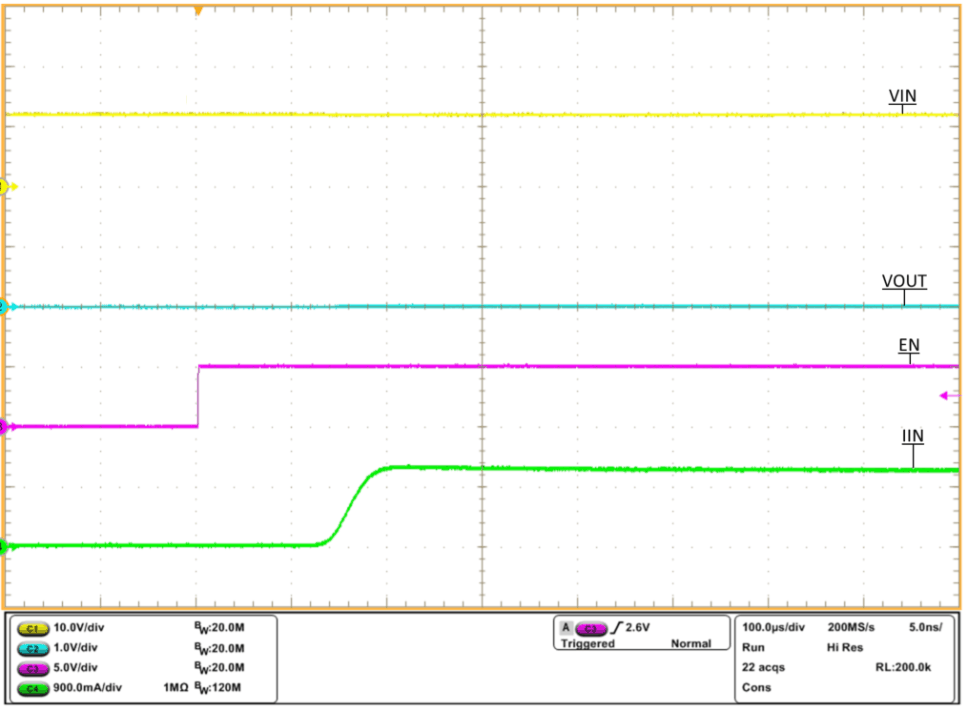VIN = 12 V, COUT = 1 µF, RILM = 487 Ω
Figure 37. Wake Up With Output Short to GND (Zoomed In)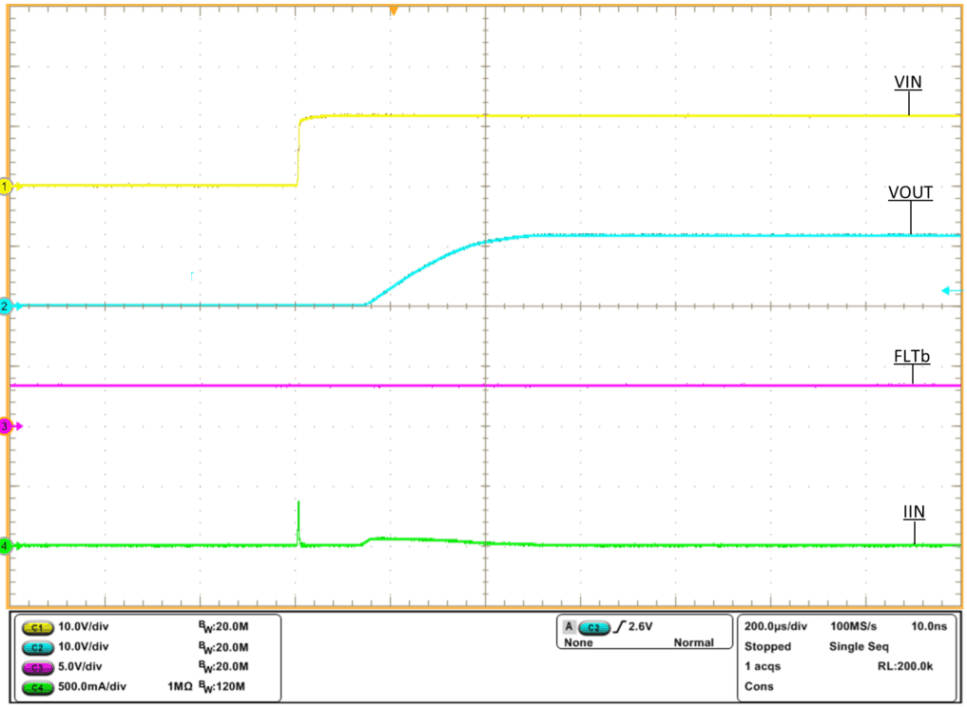VIN = 12 V, COUT = 1 µF, RILM = 487 Ω, FLT = 3.3 V through 10 kΩ
Figure 39. Hot Plug Response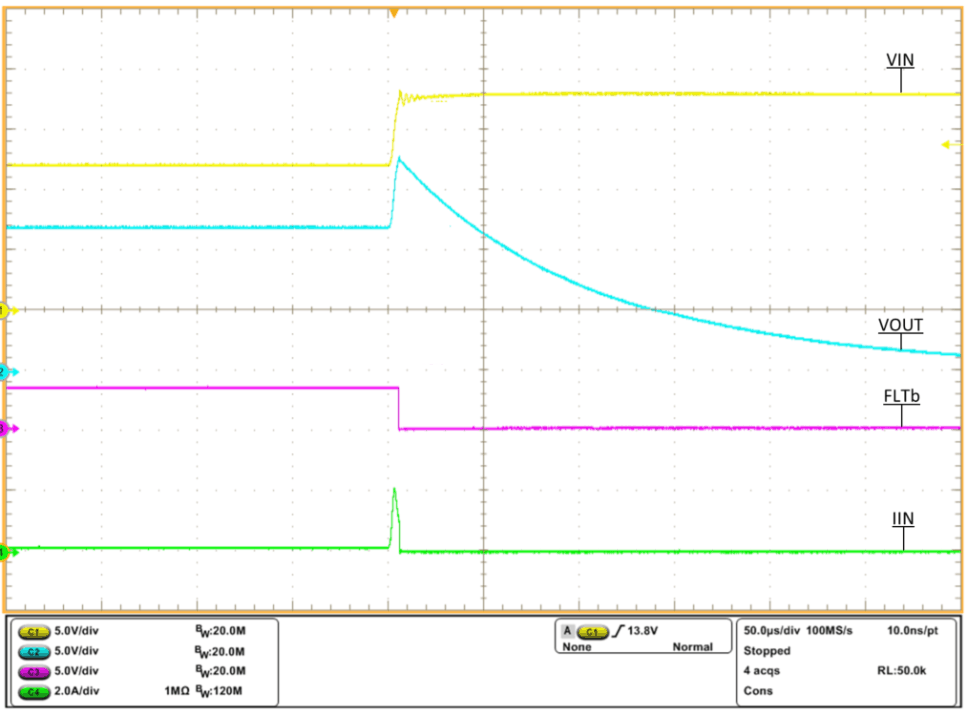VIN stepped from 12 V to 18 V, COUT = 1 µF, RILM = 487 Ω, FLT = 3.3 V through 10 kΩ, ROUT = 100 Ω
Figure 41. TPS259573 Overvoltage Lockout FLT Response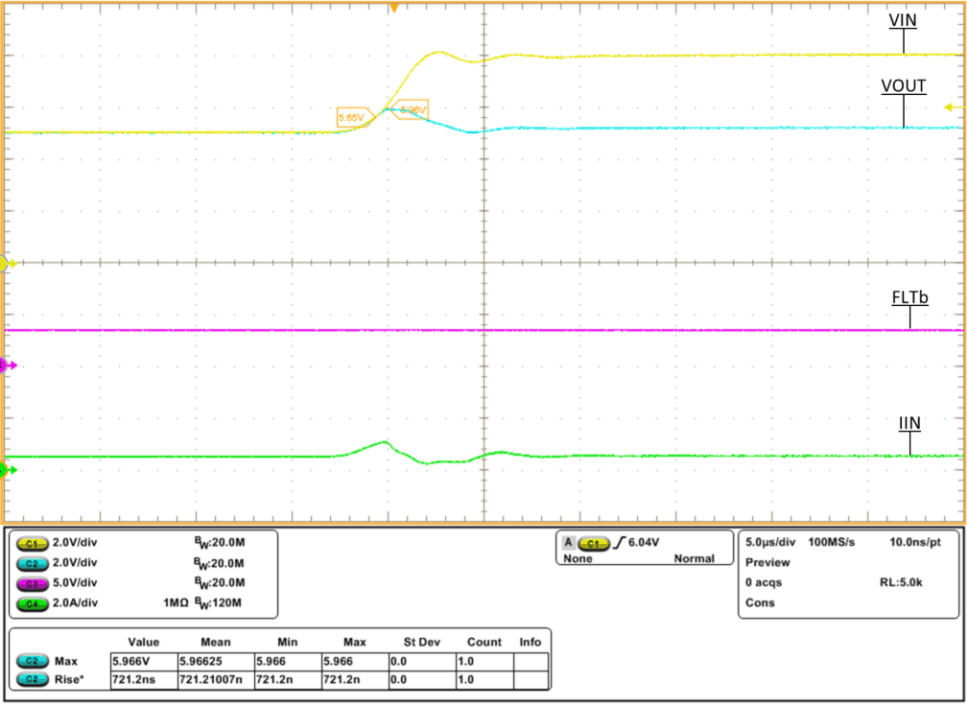VIN stepped from 5 V to 8 V, COUT = 1 µF, RILM = 487 Ω, FLT = 3.3 V through 10 kΩ, ROUT = 10 Ω
Figure 43. TPS25953x Overvoltage Clamp Response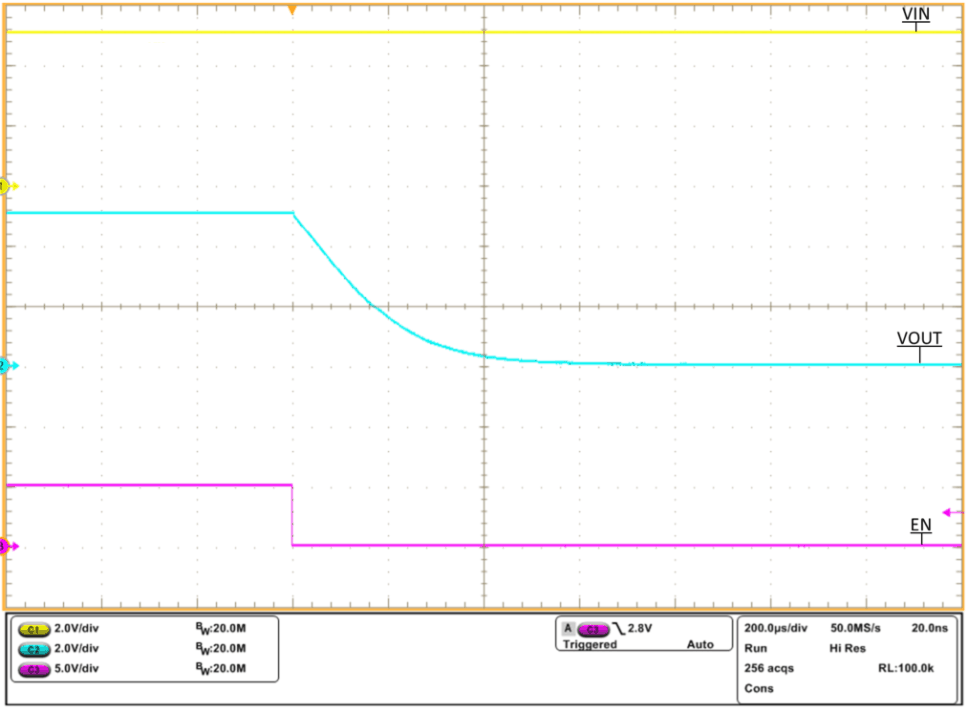VIN = 5 V, COUT = 1 µF, RILM = 487 Ω
Figure 45. TPS2595x5 Quick Output Discharge, EN stepped from 5 V to 0 V